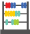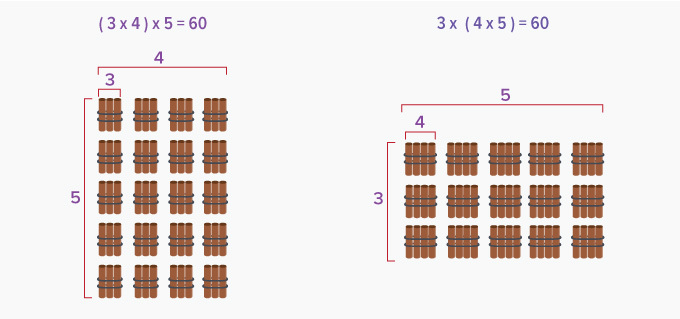# Associative Property Of Multiplication - Definition with Examples

The Complete K-5 Math Learning Program Built for Your Child

• 40 Million Kids

Loved by kids and parent worldwide

• 50,000 Schools

Trusted by teachers across schools

• Comprehensive Curriculum

Aligned to Common Core

##Let's learn!

What is the associative property of multiplication?
To “associate” means to connect or join with something.
According to the associative property of multiplication, the product of three or more numbers remains the same regardless of how the numbers are grouped.

Here’s an example of how the product does not change irrespective of how the factors are grouped.Here is another example.

 ( 7 x 8 ) x 11 = 56 x 11 = 616 7 x ( 8 x 11 ) = 7 x 88 = 616

 Fun Facts The associative property always involves 3 or more numbers. The numbers grouped within a parentheses, are terms in the expression that considered as one unit. There is also an associative property of addition. However, subtraction and division are not associative.

##Let's sing!

When multiplying numbers three or more,
Group them anyway, you will still score!
Don’t let multiplying in order, be the aim,
Because the product will just be the same!

##Let's do it!

Take your child out to a library. Ask them calculate the approximate number of books in one section of the library. Ask them to count the number of shelves, the number of racks in the section and number of books on a shelf. (Assume the number of books in each shelf is the same.) Ask the children to multiply them to find the number of books in that section. Ask them to check their calculations using the associative property of multiplication.##Related math vocabulary

Won Numerous Awards & Honors